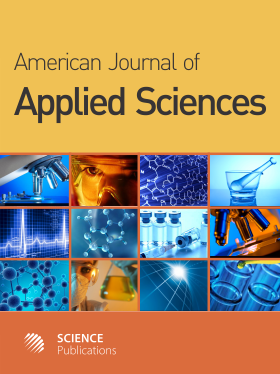Frequency: Continuous
ISSN: 1546-9239 (Print)
ISSN: 1554-3641 (Online)
Research Article Open Access

# Moisture Content Prediction of Dried Longan Aril from Dielectric Constant Using Multilayer Perceptrons and Support Vector Regression

Sanong Amaroek1, Nipon Theera-Umpon1, Kittichai Wantanajittikul1 and Sansanee Auephanwiriyakul1
• 1 ,
American Journal of Applied Sciences
Volume 7 No. 10, 2010, 1387-1392

DOI:

Submitted On: 26 September 2010 Published On: 31 October 2010

How to Cite: Amaroek, S., Theera-Umpon, N., Wantanajittikul, K. & Auephanwiriyakul, S. (2010). Moisture Content Prediction of Dried Longan Aril from Dielectric Constant Using Multilayer Perceptrons and Support Vector Regression. American Journal of Applied Sciences, 7(10), 1387-1392. https://doi.org/10.3844/ajassp.2010.1387.1392

## Abstract

Problem statement: Estimation of moisture contents of dried food products from their dielectric constants was an important step in moisture measurement systems. The regression models that provide good prediction performance are desirable. Approach: The Multilayer Perceptrons (MLP) and Support Vector Regression (SVR) were applied in this research to predict the moisture contents of dried longan arils from their dielectric constants. The data set was collected from 1500 samples of dried longan aril with five different moisture contents of 10, 14, 18, 22 and 25% Wet basis (Wb.) Dielectric constant of dried longan aril was measured by using our previously proposed electrical capacitance-based system. The results from the MLP and SVR models were compared to that from the linear regression and polynomial regression models. To take into account the generalization of the models, the four-fold cross validation was applied. Results: For the training sets, the average mean absolute errors over three bulk densities of 1.30, 1.45 and 1.60 g cm-3 were 1.7578, 0.6157, 0.3812, 0.3113, 0.0103 and 0.0044% Wb for the linear regression, second-, third-, fourth-order polynomial regression, MLP and SVR models, respectively. For the validation sets, the average mean absolute errors over the three bulk densities were 1.7616, 0.6192, 0.3844, 0.3146, 0.0126 and 0.0093% Wb for the linear regression, 2nd, 3rd and 4th-order polynomial regression, MLP and SVR models, respectively. Conclusion: The regression models based on MLP and SVR yielded better performances than the models based on linear regression and polynomial regression on both training and validation sets. The models based on MLP and SVR also provided robustness to the variation of bulk density. Not only for dried longan aril, the proposed models can also be adapted and applied to other materials or dried food products.

• 1,517 Views
• 2 Citations

### Keywords

• Dried longan aril
• dielectric constant
• moisture content
• bulk density
• regression models
• polynomial regression
• linear regression
• artificial neural network
• multilayer perceptrons
• support vector regression
• cross validation
• Dimocarpus longan